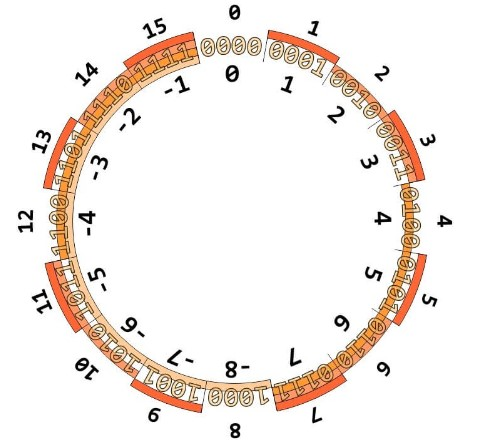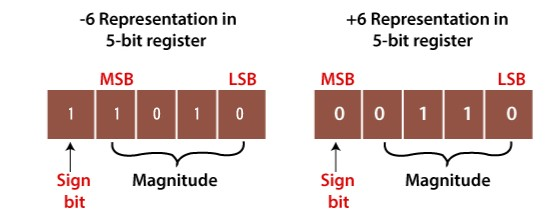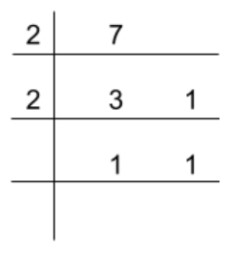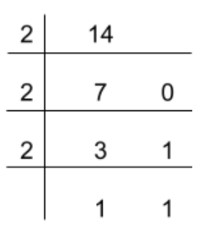# What is Two's Complement and Where do we use it?

Publish Date: 08 Aug, 2023

## Table of Content

Do you remember what integers are? Integers are a set of all the positive and negative numbers. Back in school, when we learned integers, we got to know that every number has an equal and opposite number i.e +2 and -2 e.t.c.

This is what we humans know. But computers do not understand this. And they cannot be blamed because unlike us they do not have a decimal system.

Computers understand binary numbers and in binary numbers, there are only two digits 1’s and 0’s. No negatives, no positives whatsoever.

So, how are we supposed to perform calculations including negative numbers on computers? We use one’s and two’s complements.

Both of these methods are used to represent signed numbers in computer systems. In this article, you will learn about two’s complement and where do we use it?

## What is two’s complement?

Two’s complement is a method that helps to encode negative numbers in computers. Since positive numbers are represented in the form of normal binary numbers, they do not need special representation.

Most computers use two’s complement to store signed numbers.### MSB (most significant bit):

Since two’s complement helps to represent signed numbers, it uses its MSB for this purpose. The most significant bit is the left-most bit in a binary number. For example, in 1000, 1 is the MSB.

In binary numbers, if MSB is

1. A 1 bit, then the number is a negative integer.
2. A 0 bit, then the number is a positive integer.### Sign-magnitude notation and completing a byte:

In this notation, a number is divided into two parts. One part represents the sign of the number and the other part represents magnitude.

Binary numbers already exist in the sign-magnitude notation. MSB being the 1 bit that represents the sign and the remaining n-1 bits represent the size or magnitude of binary numbers.

Many decimal numbers do not need all of the n-1 bits to represent their magnitude in binary form. For instance, 4 can be represented using only 3 bits i.e 100. So the question arises how to complete a byte (8-bits)?

In two’s complement, if the number under observation is +ve, then 0’s will be used to fill up the empty places to the left. But if the number is -ve, 1’s will be used to fill the places.

Fun Fact

Here is a little fun fact about 2’s complement.

“If a binary number is added into its two’s complement, then the number we get is 0.”

If you look carefully, it makes sense. If 4 is in the binary system then its two’s complement is -4. Adding these two, we will get 0.

## How to find two’s complement?

Two’s complement is described easily as “invert and add one”. In order to convert a binary number into two’s complement, first, we have to invert all the numbers and then add 1 to this inverted form.

Example 1 - Two's compement of a Decimal

Let’s say we want to convert 7 into two’s complement. These are the steps we will need to follow.

1. Find the binary number for 7
2. Compute its 1’s complement
3. Add 1 to find 2’s complement

Step 1: The binary number for 7.The binary number is 111. By completing the 4-bits number, we will have (0111)2

You can also use the decimal to binary converter for decimal to binary conversion.

Step 2: Calculate one’s complement of 0111.

Invert all the values. (0’s →1’s and 1’s→0’s)

= 1000

Step 3: Add 1 in 1000.

1  0 0 0

+        1

__________

1  0  0  1

Two’s complement of 7 is 1001.

Use 2’s complement calculator if you want  to skip all of the above steps for the calculation.

Example 2 -  Two's complement of a Hexadecimal

Find the 2’s complement of hexadecimal value “E”.

Solution:

The steps we will need to follow are.

1. Convert hex to decimal.
2. Find the binary number for decimal number
3. Compute 1’s complement
4. Add 1 to find 2’s complement.

Step 1: Convert hex to decimal.

E is the hexadecimal representation of decimal number 14.

Step 2: Find the binary number for 14.The binary representation for 14 is (1110)2.

Step 2: Compute one’s complement of (1110)2.

Invert all the values. (0’s →1’s and 1’s→0’s)

= 0111

Step 3: Add 1 in 0001.

0  0  0  1

+           1

__________

0  0  1  0

Two’s complement of 14 is 0010.

## Where do we use two’s complement?

As you already know, one’s complement is also used to represent signed numbers. Then why not use it instead? Why do we prefer 2’s complement to 1’s complement?

Well, the answer is for the sake of convenience. We use it for two basic reasons.

In two’s complement, adding and subtracting numbers becomes easy, similar to the method we use for decimal numbers.

While in one’s complement, adding two opposite signed numbers requires different algorithms. If you try to add them using the common method, the answer would be simply wrong.

Suppose you want to add 3 and -1. Firstly we will add using two’s complement and after that, we will try one’s complement.

In 2’s complement, 3 is represented as 1101, and -1 is represented as 1111.

1  1  0  1

+ 1  1  1  1

_____________

1   1  1  0  0

The 1 on the left is discarded due to overflow. This is 2 in two’s complement. If we convert this value into binary we will see that it is 0010. The 0 represents a positive number.

This indicates that the calculation is correct.

Now, let’s try using 1’s complement.

1  1  0  0

1  1  1  1

______________

1  1  0  1  1

The 1 on the left is discarded again. This is 4 in one’s complement. If we convert this value into binary, it will be 0100. This is the value of 4.

Hence it is proved that one’s complement cannot perform the basic arithmetic functions like addition and subtraction in a simple way.

Note: Addition using one’s complement can be done. But the method is a complex one and requires a lot of attention.

Moreover, in one’s complement, there are two representations of 0’s. One for +ve zero and one for -ve zero. This might confuse a computer. Therefore, computers use two’s complement.

Applications using one’s complement will be required to perform one extra step to make sure that the non-zero values are also non-negative zero.

### 🖩Related Tools